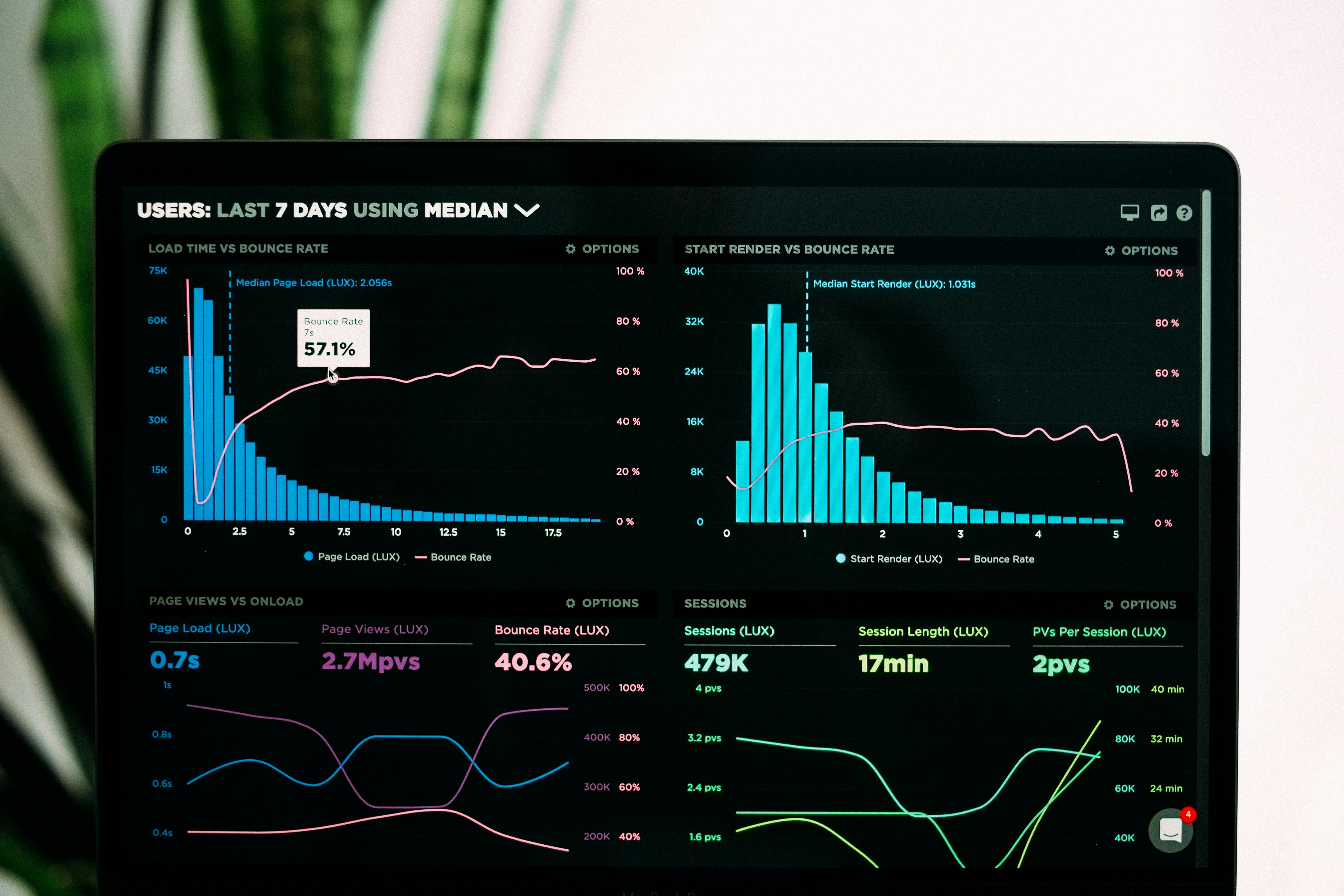# A beginners guide to Regression (Machine Learning) in PythonThis post was originally published by Bryan Dijkhuizen at Medium [AI]

## Machine Learning is making the computer learn from studying data and statistics.Machine Learning is a step into the direction of artificial intelligence (AI). Machine Learning is a program that analyses data and learns to predict the outcome.

The term regression is used when you try to find the relationship between variables. In Machine Learning and statistical modelling, that relationship is used to predict the outcome of future events.

Linear Regression uses the relationship between the data-points to draw a straight line through all them. This line can be used to predict future values.

Python has methods for finding a relationship between data-points and to draw a line of linear Regression. We will show you how to use these methods instead of going through the mathematic formula.

An example:

```x = [5,7,8,7,2,17,2,9,4,11,12,9,6]
y = [99,86,87,88,111,86,103,87,94,78,77,85,86]plt.scatter(x, y)
plt.show()```

This displays a scatter plot:

Import ‘scipy’ and draw the line of Linear Regression:

```import matplotlib.pyplot as plt
from scipy import stats```

Create the arrays that represent the values of the x and y-axis:

```x = [5,7,8,7,2,17,2,9,4,11,12,9,6]
y = [99,86,87,88,111,86,103,87,94,78,77,85,86]```

Execute a method that returns some critical fundamental values of Linear Regression:

`slope, intercept, r, p, std_err = stats.linregress(x, y)`

Create a function that uses the ‘slope’ and ‘intercept’ values to return a new deal. This new value represents where on the y-axis, the corresponding x value will be placed:

```def myfunc(x):
return slope * x + intercept```

Run each value of the x array through the function. This will result in a new collection with new values for the y-axis:

`mymodel = list(map(myfunc, x))`

Draw the original scatter plot:

`plt.scatter(x, y)`

Draw the line of linear Regression:

`plt.plot(x, mymodel)`

Display the diagram:

`plt.show()`

Multiple Regression is like linear Regression, but with more than one independent value, meaning that we try to predict a value based on two or more variables.

We can predict the CO2 emission of a car based on the size of the engine, but with multiple Regression we can throw in more variables, like the weight of the car, to make the prediction more accurate.

In Python, we have modules that will do the work for us. Start by importing the Pandas module.

`import pandas`

The Pandas module allows us to read CSV files and return a DataFrame object.

`df = pandas.read_csv("cars.csv")`

Then make a list of the independent values and call this variable x. Put the dependent values in a variable called y.

```X = df[['Weight', 'Volume']]
y = df['CO2']```

We will use some methods from the sklearn module, so we will have to import that module as well:

`from sklearn import linear_model`

From the sklearn module, we will use the ‘LinearRegression’ method to create a linear regression object.

```regr = linear_model.LinearRegression()
regr.fit(X, y)```

Now we have a regression object that is ready to predict CO2 values based on a car’s weight and volume:

`predictedCO2 = regr.predict([[2300, 1300]])`

Full Code Example

```import pandas
from sklearn import linear_modeldf = pandas.read_csv("cars.csv")X = df[['Weight', 'Volume']]
y = df['CO2']regr = linear_model.LinearRegression()
regr.fit(X, y)#predict the CO2 emission of a car where the weight is 2300kg, and the volume is 1300ccm:
predictedCO2 = regr.predict([[2300, 1300]])print(predictedCO2)```

Polynomial Regression, like linear Regression, uses the relationship between the variables x and y to find the best way to draw a line through the data points.

Python has methods for finding a relationship between data-points and to draw a line of polynomial Regression. We will show you how to use these methods instead of going through the mathematic formula. In the example below, we have registered 18 cars as they were passing a certain tollbooth.

The x-axis represents the hours of the day, and the y-axis represents the speed:

```import matplotlib.pyplot as pltx = [1,2,3,5,6,7,8,9,10,12,13,14,15,16,18,19,21,22]
y = [100,90,80,60,60,55,60,65,70,70,75,76,78,79,90,99,99,100]plt.scatter(x, y)
plt.show()```

Result

Import the modules you need:

```import numpy
import matplotlib.pyplot as plt```

Create the arrays that represent the values of the x and y-axis:

```x = [1,2,3,5,6,7,8,9,10,12,13,14,15,16,18,19,21,22]
y = [100,90,80,60,60,55,60,65,70,70,75,76,78,79,90,99,99,100]```

NumPy has a method that lets us make a polynomial model:

`mymodel = numpy.poly1d(numpy.polyfit(x, y, 3))`

Then specify how the line will display, we start at position 1, and end at position 22:

`myline = numpy.linspace(1, 22, 100)`

Draw the original scatter plot:

`plt.scatter(x, y)`

Draw the line of polynomial Regression:

`plt.plot(myline, mymodel(myline))`

Display the diagram:

`plt.show()`

It is important to know how well the relationship between the values of the x- and the y-axis is if there is no relationship the polynomial Regression can not be used to predict anything.

The relationship is measured with a value called the r-squared. The r-squared value ranges from 0 to 1, where 0 means no relationship, and one means 100% related.

How well does my data fit in a polynomial regression?

```import numpy
from sklearn.metrics import r2_scorex = [1,2,3,5,6,7,8,9,10,12,13,14,15,16,18,19,21,22]
y = [100,90,80,60,60,55,60,65,70,70,75,76,78,79,90,99,99,100]mymodel = numpy.poly1d(numpy.polyfit(x, y, 3))print(r2_score(y, mymodel(x)))```

The result 0.94 shows that there is a perfect relationship, and we can use polynomial Regression in future predictions.

Now we can use the information we have gathered to predict future values. Predict the speed of a car passing at 5 P.M:

```import numpy
from sklearn.metrics import r2_scorex = [1,2,3,5,6,7,8,9,10,12,13,14,15,16,18,19,21,22]
y = [100,90,80,60,60,55,60,65,70,70,75,76,78,79,90,99,99,100]mymodel = numpy.poly1d(numpy.polyfit(x, y, 3))speed = mymodel(17)
print(speed)```

I hope after this article you have a basic understanding of Regression and how to use it in Python and that you will be able to run yourself some scripts of Machine Learning now!